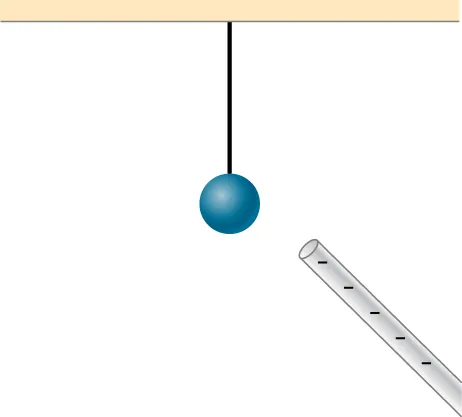University Physics Volume 2

# Conceptual Questions

University Physics Volume 2Conceptual Questions

### 5.1Electric Charge

1.

There are very large numbers of charged particles in most objects. Why, then, don’t most objects exhibit static electricity?

2.

Why do most objects tend to contain nearly equal numbers of positive and negative charges?

3.

A positively charged rod attracts a small piece of cork. (a) Can we conclude that the cork is negatively charged? (b) The rod repels another small piece of cork. Can we conclude that this piece is positively charged?

4.

Two bodies attract each other electrically. Do they both have to be charged? Answer the same question if the bodies repel one another.

5.

How would you determine whether the charge on a particular rod is positive or negative?

### 5.2Conductors, Insulators, and Charging by Induction

6.

An eccentric inventor attempts to levitate a cork ball by wrapping it with foil and placing a large negative charge on the ball and then putting a large positive charge on the ceiling of his workshop. Instead, while attempting to place a large negative charge on the ball, the foil flies off. Explain.

7.

When a glass rod is rubbed with silk, it becomes positive and the silk becomes negative—yet both attract dust. Does the dust have a third type of charge that is attracted to both positive and negative? Explain.

8.

Why does a car always attract dust right after it is polished? (Note that car wax and car tires are insulators.)

9.

Does the uncharged conductor shown below experience a net electric force?10.

While walking on a rug, a person frequently becomes charged because of the rubbing between their shoes and the rug. This charge then causes a spark and a slight shock when the person gets close to a metal object. Why are these shocks so much more common on a dry day?

11.

Compare charging by conduction to charging by induction.

12.

Small pieces of tissue are attracted to a charged comb. Soon after sticking to the comb, the pieces of tissue are repelled from it. Explain.

13.

Trucks that carry gasoline often have chains dangling from their undercarriages and brushing the ground. Why?

14.

Why do electrostatic experiments work so poorly in humid weather?

15.

Why do some clothes cling together after being removed from the clothes dryer? Does this happen if they’re still damp?

16.

Can induction be used to produce charge on an insulator?

17.

Suppose someone tells you that rubbing quartz with cotton cloth produces a third kind of charge on the quartz. Describe what you might do to test this claim.

18.

A handheld copper rod does not acquire a charge when you rub it with a cloth. Explain why.

19.

Suppose you place a charge q near a large metal plate. (a) If q is attracted to the plate, is the plate necessarily charged? (b) If q is repelled by the plate, is the plate necessarily charged?

### 5.3Coulomb's Law

20.

Would defining the charge on an electron to be positive have any effect on Coulomb’s law?

21.

An atomic nucleus contains positively charged protons and uncharged neutrons. Since nuclei do stay together, what must we conclude about the forces between these nuclear particles?

22.

Is the force between two fixed charges influenced by the presence of other charges?

### 5.4Electric Field

23.

When measuring an electric field, could we use a negative rather than a positive test charge?

24.

During fair weather, the electric field due to the net charge on Earth points downward. Is Earth charged positively or negatively?

25.

If the electric field at a point on the line between two charges is zero, what do you know about the charges?

26.

Two charges lie along the x-axis. Is it true that the net electric field always vanishes at some point (other than infinity) along the x-axis?

### 5.5Calculating Electric Fields of Charge Distributions

27.

Give a plausible argument as to why the electric field outside an infinite charged sheet is constant.

28.

Compare the electric fields of an infinite sheet of charge, an infinite, charged conducting plate, and infinite, oppositely charged parallel plates.

29.

Describe the electric fields of an infinite charged plate and of two infinite, charged parallel plates in terms of the electric field of an infinite sheet of charge.

30.

A negative charge is placed at the center of a ring of uniform positive charge. What is the motion (if any) of the charge? What if the charge were placed at a point on the axis of the ring other than the center?

### 5.6Electric Field Lines

31.

If a point charge is released from rest in a uniform electric field, will it follow a field line? Will it do so if the electric field is not uniform?

32.

Under what conditions, if any, will the trajectory of a charged particle not follow a field line?

33.

How would you experimentally distinguish an electric field from a gravitational field?

34.

A representation of an electric field shows 10 field lines perpendicular to a square plate. How many field lines should pass perpendicularly through the plate to depict a field with twice the magnitude?

35.

What is the ratio of the number of electric field lines leaving a charge 10q and a charge q?

### 5.7Electric Dipoles

36.

What are the stable orientation(s) for a dipole in an external electric field? What happens if the dipole is slightly perturbed from these orientations?

Order a print copy

As an Amazon Associate we earn from qualifying purchases.